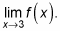##### Pre-Calculus: 1001 Practice Problems For Dummies (+ Free Online Practice)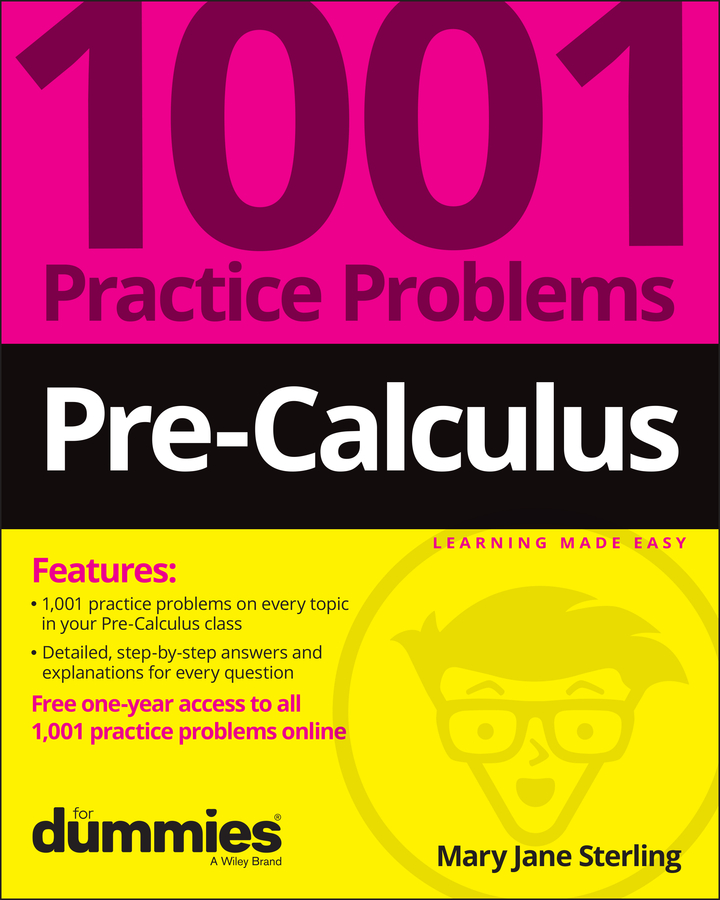When you’re given the graph of a function and your pre-calculus teacher asks you to find the limit, you read values from the graph — something you’ve been doing ever since you learned what a graph was! If you’re looking for a limit from the left, you follow that function from the left-hand side toward the x value in question. Repeat this process from the right to find the right-hand limit. If the y value being approached from the left is the same as the y-value being approached from the right (did the pencils meet?), that y value is the limit. Because the process of graphing a function can be long and complicated, you shouldn’t use the graphing approach unless you’ve been given the graph.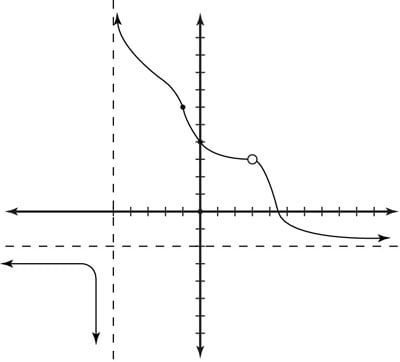Finding the limit of a function graphically.

For example, find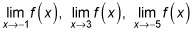in the preceding figure.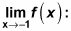You can see that as the x-value gets closer and closer to –1, the value of the function f(x) approaches 6. And in fact, when x gets to –1, the function’s value actually is 6! Technically, though, having f(–1) = 6 isn’t required in order to say that the limit is 6; the function could have a hole in it at that point, and the limit would still be 6! See the next example: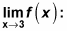In the graph, you can see a hole in the function at x = 3, which means that the function is undefined — but that doesn’t mean you can’t state a limit. If you look at the function’s values from the left,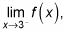and from the right,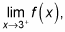you see that the y value approaches 3. So you say that the limit of the function as x approaches 3 is 3.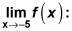You can see that the function has a vertical asymptote at x = –5. From the left, the function approaches negative infinity as it nears x = –5. You can express this mathematically as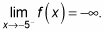From the right, the function approaches infinity as it nears x = –5. You write this situation as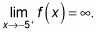Therefore, the limit doesn’t exist at this value, because the left-hand limit is negative infinity but the right-hand limit is infinity.

For a function to have a limit, the left and right limits must be the same. A function can have a hole in the graph at a particular x value but the limit as x approaches this value can still exist, like